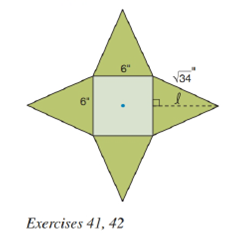Chapter 9.2, Problem 41EElementary Geometry For College St...

7th Edition
Alexander + 2 others
ISBN: 9781337614085

Solutions

Chapter
SectionElementary Geometry For College St...

7th Edition
Alexander + 2 others
ISBN: 9781337614085
Textbook Problem

Consider the accompanying figure. When the four congruent isosceles triangles are folded upward, a regular square pyramid is formed. What is the surface area (total area) of the pyramid?To determine

To find:

The surface area (total area) of the pyramid.

Explanation

Given:

The four congruent isosceles triangles are folded upward it forms a regular square pyramid and the figure given below

Properties Used:

The total surface area T of a pyramid with lateral area L and base area B is given by

T=L+B.

The lateral area L of a regular pyramid with slant height of length l and perimeter P of the base is given by

L=12lP.

According to the Pythagorean theorem, in a right-angled triangle

hypotenuse2=base2+perpendicular2.

The area of the square is side2.

Approach Used:

i) Find the slant height in the right-angled triangle by applying Pythagoras Theorem.

ii) Calculate the perimeter of the base P.

iii) Substitute the values of P and the given lateral area in equation L=12lP to find the value of slant height l.

iv) Calculate the base area of the square base formed.

v) Add the two areas from step (iii) and step (iv) to get the total surface area.

Calculation:

From the figure below,

considering one of the lateral faces which is an isosceles triangle

Still sussing out bartleby?

Check out a sample textbook solution.

See a sample solution

The Solution to Your Study Problems

Bartleby provides explanations to thousands of textbook problems written by our experts, many with advanced degrees!

Get Started

Find more solutions based on key concepts